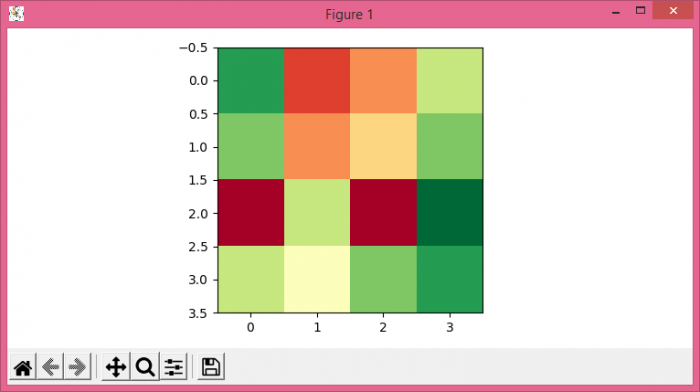# How do you improve Matplotlib image quality?

To improve matplotlib image quality we can use greater dot per inch i.e dpi value (greater than 600) and pdf or .eps format can be recommended.

## Steps

• Set the figure size and adjust the padding between and around the subplots.
• Make a 2D data raster using a np.array.
• Display data as an image, i.e., on a 2D regular raster.
• Save the current image using savefig() with dpi=1200 and .eps format,
• To display the figure, use show() method.

## Example

import numpy as np
from matplotlib import pyplot as plt
plt.rcParams["figure.figsize"] = [7.50, 3.50]
plt.rcParams["figure.autolayout"] = True
data = np.array(
[[0.1, 0.7, 0.6, 0.3],
[0.2, 0.6, 0.5, 0.2],
[0.8, 0.3, 0.80, 0.01],
[0.3, 0.4, 0.2, 0.1]]
)
plt.imshow(data, interpolation="nearest", cmap="RdYlGn_r")
plt.savefig("myimage.eps", dpi=1200)
plt.show()

## Output